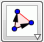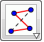﻿ Polygon

##Rigid Polygon

A Rigid Polygon is a polygon that can only be rotated and translated. Its shape cannot be altered.

It is drawn in the same way as an ordinary polygon: the tool is selected and then at least three points which will be the vertices of the polygon must be selected and then the first point is clicked again.

##Vector Polygon

A Vector Polygon is a polygon where the first point presents the origin (the position of the other points is calculated according to this point).

It can be drawn in the same way as a Rigid Polygon.

##PolyLine

With the PolyLine tool a polygonal line can be drawn.

Video presentation for the tools Rigid Polygon, Vector Polygon and PolyLine.

Source files for video presentation.

Main page